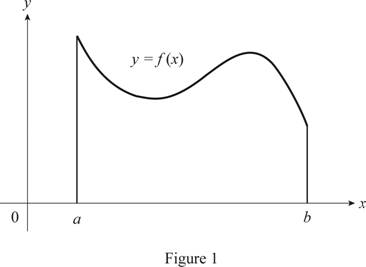# The definition for the definite integral of a continuous function from a to b .### Single Variable Calculus: Concepts...

4th Edition
James Stewart
Publisher: Cengage Learning
ISBN: 9781337687805### Single Variable Calculus: Concepts...

4th Edition
James Stewart
Publisher: Cengage Learning
ISBN: 9781337687805

#### Solutions

Chapter 5, Problem 2RCC

(a)

To determine

## To write: The definition for the definite integral of a continuous function from a to b.

Expert Solution

### Explanation of Solution

Consider the function f which is continuous on interval [a,b].

Divide the interval into n subintervals of equal width, Δx=ban.

Let x0(=a),x1,x2,....,xn(=b) be the end and intermediate points of the subintervals.

Then, the definite interval is the sum of the area of the subintervals. It is expressed as follows:

abf(x)dx=limni=1nf(xi*)Δx

Here, xi* is any sample point in the ith subinterval [xi1,xi].

(b)

To determine

Expert Solution

### Explanation of Solution

The function f(x)0 represents the function in the first quadrant of the graph.

Sketch the curve f(x) in the first quadrantas shown in Figure 1.From Figure 1, the function f is positive when f0.

The geometric interpretation of abf(x)dx refers that the total area which is the region under the graph y=f(x) and above the x-axis on the interval [a,b].

(c)

To determine

Expert Solution

### Explanation of Solution

The function f(x) consists both positive and negative values which means that the graph of y=f(x) forms the region above and below the x-axis.

The graph of function y=f(x) for both positive and negative value of f(x) is shown in the below Figure 1.From the definition from part (a), as f(x) takes both positive and negative values the limit of each Riemann sum is also positive, negative or zero.

Thus, the geometric interpretation of abf(x)dx refers the signed area under the graph y=f(x) above the x-axis and below the x-axis on the interval [a,b].

### Have a homework question?

Subscribe to bartleby learn! Ask subject matter experts 30 homework questions each month. Plus, you’ll have access to millions of step-by-step textbook answers!